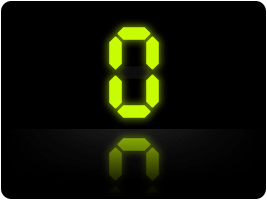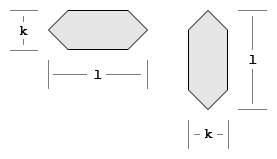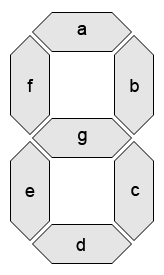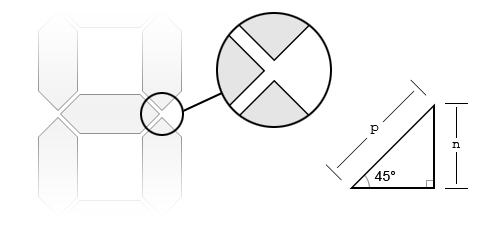# Simulate a Seven-Segment Display in Flash (AS3)

Seven-segment displays are widely used in electronic and mechanical devices to display simple information such as the time (a digital clock) or a price (a local gas station's price per gallon). Advantages include ease of implementation, updating, and the most important: cost. With only 7 segments, such as a LED, you can display 10 different numerals (ie 0-9).

In the software design world, seven-segment displays can be useful because they are a fixed width, people recognize them and they have an interesting look. In this tutorial I will give explain the math behind a seven-segment display how to implement them in Flash ActionScript 3 (AS3).## What is a Seven-Segment Display?

Seven-segment displays (7-seg displays) are an effective and low-cost solution for displaying the numerals: 0,1,2,3,4,5,6,7,8,9. Multiple 7-seg displays placed in a row provides the ability to display larger numbers. With additional glyphs such as a colon more opportunites present themselves, such as displaying the time.

Segment displays come in a few different types including: Nine-segment, Fourteen-segment, and Sixteen-segment. Seven-segment are covered in this article. The decimal point usually included with segment displays will not be ignored. Also note that 7-segs are usually in italics (for readability and an interesting trick which requires multiple displays) but for simplicity no leans for my glpyhs.

## The Segment

The building blocks of a segment display are its segments. The properties of a simple segment are:

• segment thickness (k): thickness of a segment
• segment length (l): length of a segment
• segment ratio (r): the segment thickness divided by the width k/l
• segment orientation (o): either horizontal or vertical## The Digit

Seven segments can create a digit. The segments are labeled "a" through "g" in a clockwise fashion:The major variable which will effect our digit is the padding between segments defined as padding (p). However computer languages are based in the Cartesian coordinate system so we will need to convert this variable to a new variable which we will call: segmentPaddingCartesian (n).

To do this we focus on the space between our segment ends and find an isosceles right triangle.Using a little trigonometry:

• n/p = sin(45°)
• n = sin(45°)*p
• n = (√2)/2*p

We can define segmentPaddingCartesian (n) as (√2)/2 multiplied by the padding (p).

## The Glyphs

Although a seven-segment displays can display a number of glyphs our digit will only display the ten numerals: 0 - 9. The following truth table outlines which segments should be on or off to represent each glyph:

a b c d e f g
0 1 1 1 1 1 1 0
1 0 1 1 0 0 0 0
2 1 1 0 1 1 0 1
3 1 1 1 1 0 0 1
4 0 1 1 0 0 1 1
5 1 0 1 1 0 1 1
6 1 0 1 1 1 1 1
7 1 1 1 0 0 0 0
8 1 1 1 1 1 1 1
9 1 1 1 1 0 1 1Figure 1: Glyph Truth Table

## Psudeocode

Before getting language specific here is the pseudocode:

```createDigit()

function createDigit()
for each segment (A-G)
if (segment width is greater than segment height)
draw segment horizontal
else
draw segment vertical

function updateDigit(glyph)
if (glyph is not valid)
throw error
return
else
for each segment (A-G)
if (glyph array with glyph index is true)
make segment visible
else
make segment hidden```

## AS3 Implementation

First we need to define the state of each segment for each glyph. We achieve this through a multi-dimensional array called 'glyphs'. For example for the first glyph '0', all the segment states are true except the last segment.

`glyphs = new Array(true, true, true, true, true, true, false);`

We continue the define the rest of the glyphs (1-9):

```glyphs = new Array(false, true, true, false, false, false, false);
glyphs = new Array(true, true, false, true, true, false, true);
glyphs = new Array(true, true, true, true, false, false, true);
glyphs = new Array(false, true, true, false, false, true, true);
glyphs = new Array(true, false, true, true, false, true, true);
glyphs = new Array(true, false, true, true, true, true, true);
glyphs = new Array(true, true, true, false, false, false, false);
glyphs = new Array(true, true, true, true, true, true, true);
glyphs = new Array(true, true, true, true, false, true, true);```

Next we create a segment object for each segment. We do this now, because we are about to assign an angle to each segment.

```// For each segment (a-g)
var i:int = A;
for(; i <= G + 1; i++)
{
segments[i] = new Object();
}```

Now we set the angle of each segment to either 0 (horizontal) or 90 (vertical):

```// Define each segment's angle
segments[A].angle = 0;
segments[B].angle = 90;
segments[C].angle = 90;
segments[D].angle = 0;
segments[E].angle = 90;
segments[F].angle = 90;
segments[G].angle = 0;```

For each segment we create a shape object so we can draw the graphics.

```// For each segment (a-g)
for(i = A; i <= G; i++)
{
segments[i].container = new Shape();
}```

Now that the object is created, we will need to update it as properties change (ie a change in the value or the height). Let's put it into a seperate method so we can call it when need it. The first part of this method updates properties.

```// Update some variables that may have changed
digitWidth = digitHeight / 2;
segmentThickness = digitHeight * thicknessRatio;
segmentLength = segmentThickness / segmentRatio;

Since a property may have changed, we need to redefine each segment's position.

```// Define each segment's position
segments[A].x = segmentThickness / 2 + segmentPaddingCartesian;
segments[A].y = 0;

segments[B].x = segments[A].x + segmentLength - segmentThickness / 2 + segmentPaddingCartesian;
segments[B].y = segments[A].x;

segments[C].x = segments[B].x;
segments[C].y = segments[B].y + segmentLength + 2 * segmentPaddingCartesian;

segments[D].x = segments[A].x;
segments[D].y = segments[C].y + segmentLength - segmentThickness / 2 + segmentPaddingCartesian;

segments[E].x = 0;
segments[E].y = segments[C].y;

segments[F].x = 0;
segments[F].y = segments[B].y;

segments[G].x = segments[A].x;
segments[G].y = segments[B].y + segmentLength - segmentThickness / 2 + segmentPaddingCartesian;```

Now we position and draw each glpyh:

```// For each segment (a-g)
var i:int = A;
for(; i <= G; i++)
{
// Position each segment
segments[i].container.x = segments[i].x;
segments[i].container.y = segments[i].y;

// Draw the segment
segments[i].container.graphics.clear();
segments[i].container.graphics.beginFill(segmentColor);
if(!segments[i].angle)
{
segments[i].container.graphics.moveTo(0, segmentThickness / 2);

segments[i].container.graphics.lineTo(segmentThickness / 2, 0);
segments[i].container.graphics.lineTo(segmentLength - segmentThickness / 2, 0);
segments[i].container.graphics.lineTo(segmentLength, segmentThickness / 2);
segments[i].container.graphics.lineTo(segmentLength - segmentThickness / 2, segmentThickness);
segments[i].container.graphics.lineTo(segmentThickness / 2, segmentThickness);
segments[i].container.graphics.lineTo(0, segmentThickness / 2);
}
else
{
segments[i].container.graphics.moveTo(segmentThickness / 2, 0);

segments[i].container.graphics.lineTo(0, segmentThickness / 2);
segments[i].container.graphics.lineTo(0, segmentLength - segmentThickness / 2);
segments[i].container.graphics.lineTo(segmentThickness / 2, segmentLength);
segments[i].container.graphics.lineTo(segmentThickness, segmentLength - segmentThickness / 2);
segments[i].container.graphics.lineTo(segmentThickness, segmentThickness / 2);
segments[i].container.graphics.lineTo(segmentThickness / 2, 0);
}
segments[i].container.graphics.endFill();
}```

The digit class includes a couple more methods (ie addParent and updateValue) and properties including color, value, and height. Hopefully these are self-explanatory.

## AS3 Example

Selecting a choice from the combo box will set the value property of the digit object.

As an example a simple web interface that imports the digit class and provides a combo box to change the digit via the value method is made. Full documentation of the digit class can be found here.

The following code instantiates the digit object and sets some default properties.

```import com.williammalone.Digit;

var digit:Digit = new Digit();
digit.height = 100;
digit.x = 240;
digit.y = 20;
digit.color = 0x557755;

We create a combo box that will change the value properties of the digit object.

```import fl.controls.ComboBox;

var changeDigit:ComboBox = new ComboBox();
changeDigit.width = 120;
changeDigit.x = 40;
changeDigit.y = 10;
changeDigit.prompt = "Select a digit";
changeDigit.addItem( { label: "0", data:0 } );
changeDigit.addItem( { label: "1", data:1 } );
changeDigit.addItem( { label: "2", data:2 } );
changeDigit.addItem( { label: "3", data:3 } );
changeDigit.addItem( { label: "4", data:4 } );
changeDigit.addItem( { label: "5", data:5 } );
changeDigit.addItem( { label: "6", data:6 } );
changeDigit.addItem( { label: "7", data:7 } );
changeDigit.addItem( { label: "8", data:8 } );
changeDigit.addItem( { label: "9", data:9 } );